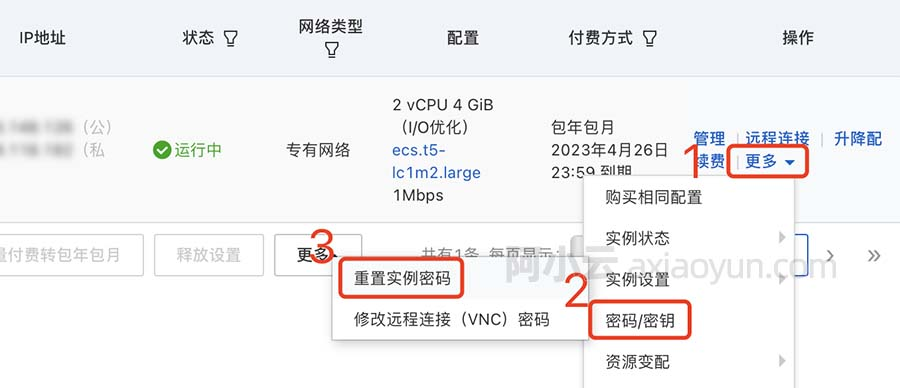# word2vec深入浅出，保证你一看就会（3）

+关注继续查看

## Hierarchical Softmax$$p(n, left) = σ（v_i^Th）$$

，往右是

$$1-σ（v_i^Th）$$

forward

$$p=σ（[*]n(w, 1)^Th)*σ（[*]n(w, 2)^Th)....其中[*]表示向左走取+1，向右走取-1$$

backward

$$E=loss=-log p$$

$$\frac{\partial E}{\partial v_i^Th}=σ( v_i^Th)-t_j$$

$$[*]=+1时,t_j=1,[*]=-1时,t_j=0$$

$$\frac{\partial E}{\partial v_i}=(σ( v_i^Th)-t_j)*h$$

## 代码分析

for (d = 0; d < vocab[word].codelen; d++) {
f = 0;
l2 = vocab[word].point[d] * layer1_size;
// Propagate hidden -> output
for (c = 0; c < layer1_size; c++) f += neu1e[c] * syn1[c + l2];
g= 输出层错误 \partial E / \partial v_i^T*h
// Learn weights hidden -> output
for (c = 0; c < layer1_size; c++) syn1[c + l2] += g * neu1e[c];
}

syn1 ：在前一篇文章中也提到 是$$w2$$即现在上图中的黑色节点的集合，然后把两维拉成了一维。
l2 = vocab[word].point[d] * layer1_size 计算了这个词每个经过节点在syn1数组中的开始地址。

$$\frac{\partial E}{\partial v_i}=(σ( v_i^Th)-t_j)*h$$

  for (c = 0; c < layer1_size; c++) f += neu1e[c] * syn1[c + l2];

g= 输出层错误 \partial E / \partial v_i^T*h

 for (c = 0; c < layer1_size; c++) syn1[c + l2] += g*neu1e[c];

neu1e即h。符合上面公式的推导。

## Hierarchical Softmax 讨论3213 04558 0Xshell使用SSH远程登录阿里云ECS服务器CentOS7
10242 08045 09476 0word2vec深入浅出，保证你一看就会（2）

3738 0word2vec深入浅出，保证你一看就会（5）【完结】

3015 0652 02368 04051 0
6

0

《SaaS模式云原生数据仓库应用场景实践》

《看见新力量：二》电子书